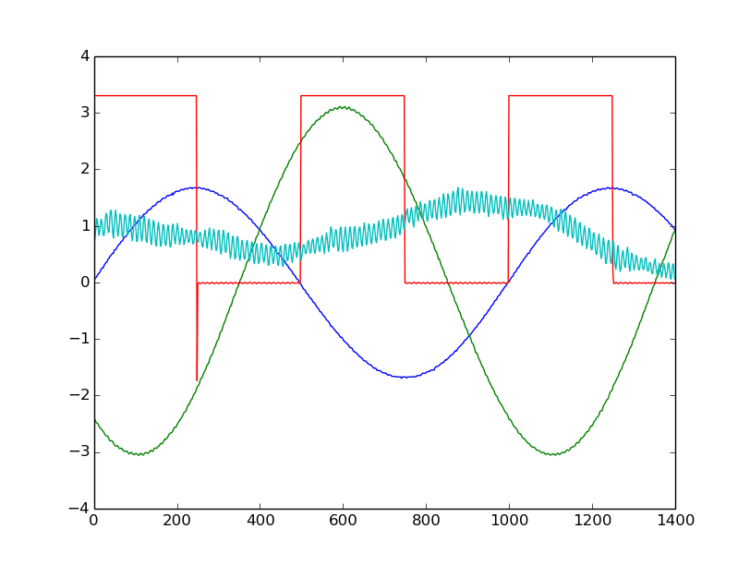## Communicating with Pocket Science Lab via USB and capturing and plotting sine waves

Design of PSLab combines the flexibility of Python programming language and the real-time measurement capability of micro-controllers.

PSLab, with its simple and open architecture allows users to use the tool for various measurements and to develop new experiments with simple functions written in python.

PSLab is interfaced and powered by USB port of the computer. For connecting external signals it has several input/output terminals as shown in the figure.#### Interfacing with the real world

Connecting to PSLab is as simple and straight forward as this…

```>>> from PSL import sciencelab
>>> I = sciencelab.connect()     #Returns None if device isn't found
# An example function that measures voltage present at the specified analog input
>>> print I.get_average_voltage('CH1')```

Various sensors can be connected to PSLab and data can be fetched with a simple python code as shown below…

```>>> from PSL.SENSORS import HMC5883L #A 3-axis magnetometer
>>> M = HMC5883L.connect()
>>> Gx,Gy,Gz = M.getRaw()
```

The module sciencelab.py contains all the functions required for communicating with PSLab hardware. It also contains some utility functions. The class ScienceLab() contains methods that can be used to interact with the PSLab. The connect() function returns an object of this class if PSLab hardware is detected.

The initialization process does the following

* connects to tty device

```>>> from PSL import sciencelab
>>> I = sciencelab.connect()
>>> print I
<PSL.sciencelab.ScienceLab instance at 0x7fe9a7bf0e18>```

After initiating this class, its various function calls will allow access to all the features built into the device. Some examples showing the use of few function calls are given below…

#### Example 1: Capturing and plotting a sine wave

The function call used,

`capture1(self,ch,ns,tg,*args,**kwargs)`

Arguments

• ch  : Channel to select as input. [‘CH1′..’CH3′,’SEN’]
• ns  :  Number of samples to fetch. Maximum 10000
• tg   :  Time gap between samples in microseconds

Example Program

Connect WG1 to CH1 and run the following code.

```>>> from pylab import *
>>> from PSL import sciencelab
>>> I=sciencelab.connect()
>>> I.set_gain('CH1', 3) # set input CH1 to +/-4V range
>>> I.set_sine1(1000) # generate 1kHz sine wave on output W1
>>> x,y = I.capture1('CH1', 1000, 10) # digitize CH1 1000 times, with 10 usec interval
>>> plot(x,y)
>>> show()```

For running the script in IDE, one should define source code encoding, add this to the top of your script:

``# -*- coding: utf-8 -*-``

The output of the program is here…#### Example 2 : Capturing two sine waves and plotting

The function call used,

`capture2(self,ns,tg,TraceOneRemap='CH1')`

Arguments

• ns :  Number of samples to fetch. Maximum 5000
• tg  :  Time gap between samples in microseconds
• TraceOneRemap :   Choose the analogue input for channel 1 (Like MIC OR SEN). It is connected to CH1 by default. Channel 2 always reads CH2.

Example Program

Connect WG1 to CH1, WG2 to CH2 and run the following code.

```# -*- coding: utf-8 -*-

from pylab import *
from PSL import sciencelab
I=sciencelab.connect()
I.set_gain('CH1', 2) # set input CH1 to +/-4V range
I.set_gain('CH2', 3) # set input CH2 to +/-4V range
I.set_sine1(1000) # generate 1kHz sine wave on output W1
I.set_sine2(1000) # generate 1kHz sine wave on output W2

x,y1,y2 = I.capture2(1600,1.75,'CH1')
show()```

The output of the program is here…#### Example 3 : Capturing four traces and plotting

The function call used,

`capture4(self,ns,tg,TraceOneRemap='CH1')`

Arguments

• ns:   Number of samples to fetch. Maximum 2500
• tg :   Time gap between samples in microseconds. Minimum 1.75uS
• TraceOneRemap :   Choose the analogue input for channel 1 (Like MIC OR SEN). It is connected to CH1 by default. Channel 2 always reads CH2.

Example Program

Connect WG1 to CH1, WG2 to CH2, SQR1 to CH3 and transducer mic to MIC (CH4) and run the following code.

```# -*- coding: utf-8 -*-

from pylab import *
from PSL import sciencelab
I=sciencelab.connect()
I.set_gain('CH1', 2) # set input CH1 to +/-4V range
I.set_gain('CH2', 3) # set input CH2 to +/-4V range
I.set_sine1(1000) # generate 1kHz sine wave on output W1
I.set_sine2(1000) # generate 1kHz sine wave on output W2
I.sqr1(2000,duty_cycle=50) # generate 1kHz square wave on output SQR1

x,y1,y2,y3,y4 = I.capture4(800,1.75)
The output of the program is here…